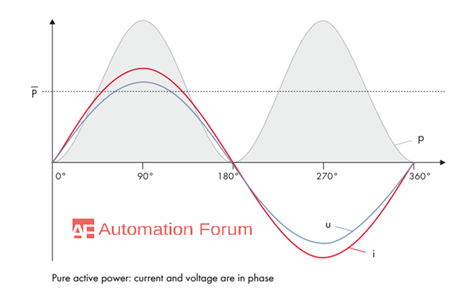# What is active and reactive power and what are the difference between them? What are the types of reactive power

What is active and reactive power

The flow of energy in an electrical power system can be described by the active and the reactive power active power is the real power and the reactive power is the power which is used for the transmission of the real power, active power is the power which is utilized by AC circuit and its measurement is in kilowatt or megawatt, it is the real outcome of an electrical system. The power which is actually consumed or utilized in an AC circuit is called true power or active power it is measured in kilowatt or in megawatt, it is the actual outcomes of the electrical system which runs the electrical circuits or loads. The power which flows back and forth which means it moves in both the direction in the circuit or reacts upon itself is called reactive power. Inductors or capacitors do not consume any power. In a half-cycle whatever power which is received from the source by these components, the same power is returned to the source. So the power which bounces back and forth between source and load is known as reactive power.

In an AC circuit, the voltage and the current are sinusoidal which means that the waveform is in a sine wave, the voltage and the current will reverse their polarity at the same time at every instant. Active power is the power that is consumed by an AC circuit the dissipated power is called as active power and is denoted by “P”The strength and the direction of both the current flow and the voltage changes periodically, the current and voltage will reach their maximum and minimum values with no phase shift, the power oscillates between the zero and the positive maximum value and by this only positive power value and only active power is generated and the active power is the usable power, which is present in the utility grid During a phase shift of 90 degrees, the current will be maximum when the voltage crosses zero and the power will oscillate between the positive and the negative values and its average value will be zero over time and this is known as reactive power ‘Q’.

What is the apparent power

Apparent power is the multiplication of current and voltage magnitudes. This can be considered as the vector sum of the circuit’s active and reactive power. AC load apparent power can be determined from the product of a voltmeter and an ammeter reading. Reactive power can be determined by vector subtraction. Total power in an AC circuit, both dissipated and absorbed or returned is referred to as apparent power. it is the combination of reactive power and true power.

What is real power and reactive power

Real power is the rate at which a load does work or dissipates energy by heat transfer and this shows the energy loss from the supply. In the case of reactive power, it can be considered as the stored energy rate in the loads magnetic or electric field and it would return to the supply every quarter cycle.

Demand for reactive power

Reactive power arises if there is a production of electric current in large power plants, and it will burden the utility grid by not contributing to the energy transport so in order to stabilize the utility grid the reactive power compensation must be done.

What are the types of reactive power

Displacement reactive power – This is caused due to the due to the displacement of the angle between current and voltage. This can be compensated by using reactor protected capacitors.

Distortion reactive power – Due to the harmonics in current and voltage this has occurred. It is provided when connecting high-performance current converters. Compensation is done by using filter circuits.

Modulation reactive power – Due to the periodic load fluctuations this type of reactive power has occurred. It is caused while connecting high power welding machines, arcing, furnaces… and compensation is done through dynamic compensation.

Asymmetric reactive power – This is caused by single and two-phase loads. It is formed when connecting high power converters such as welding machines and electric railways.

What are the difference between active and reactive power

• Active power is consumed by the load and the reactive power is useless

• Active power is the product of current and the voltage and the cosine of the angle between them in case of reactive power it is also the product of voltage and current and the sine of the angle between them

• Reactive power is measured in VAR while the active power is measured in watts

• The light in the lamps and the torque in the motor all are produced by the active power and the reactive power determines the power factor of the circuit